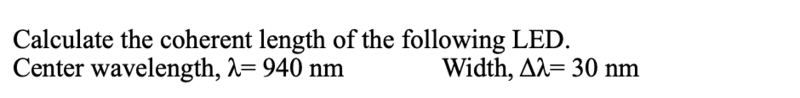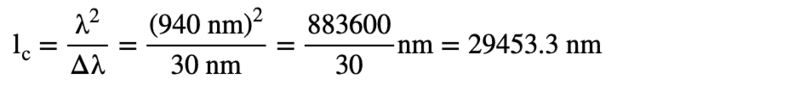# Calculate the coherent length of this LED

nao113
Homework Statement:
Calculate the coherent length of the following LED.
Center wavelength, λ= 940 nm Width, Δλ= 30 nm
Relevant Equations:
I put the equation in the picture belowHomework Helper
Gold Member
2022 Award
Homework Statement:: Calculate the coherent length of the following LED.
Center wavelength, λ= 940 nm Width, Δλ= 30 nm
Relevant Equations:: I put the equation in the picture below

View attachment 302561
View attachment 302562
Hi @nao113. You haven't actually asked a question! But a few thoughts anyway….

Is Δλ the FWHM (full width at half maximum) or the half-width? Let’s assume it is the FWHM.

The coherence (not ‘coherent’) length, L, In a medium of refractive index 1 is given by:
##L = C \frac {λ^2}{Δλ}##
e.g. see https://en.wikipedia.org/wiki/Coherence_length

You have taken C=1 but typically C≈ ½. See above link.

You have been given data to 2 significant figures but your final answer has 6 significant figures - lose 1 mark in an exam’!

•berkeman
nao113
Hi @nao113. You haven't actually asked a question! But a few thoughts anyway….

Is Δλ the FWHM (full width at half maximum) or the half-width? Let’s assume it is the FWHM.

The coherence (not ‘coherent’) length, L, In a medium of refractive index 1 is given by:
##L = C \frac {λ^2}{Δλ}##
e.g. see https://en.wikipedia.org/wiki/Coherence_length

You have taken C=1 but typically C≈ ½. See above link.

You have been given data to 2 significant figures but your final answer has 6 significant figures - lose 1 mark in an exam’!
so the answer will be 14726.7 nm?
I got the reference from my class material like this pic

#### Attachments

•Delta2
Homework Helper
Gold Member
2022 Award
so the answer will be 14726.7 nm?
That would still lose a mark (even if correct) due to excess significant figures!

However, from your attachment it appears that in your course, you are:
- using Δλ to mean the FWHM;
- using C=1 in the equation ##L = C \frac {λ^2}{Δλ }## (technically not correct, but never mind).

So, based on your course material, your original answer (in Post #1) would be correct if you rounded it properly.

•Delta2
nao113
That would still lose a mark (even if correct) due to excess significant figures!

However, from your attachment it appears that in your course, you are:
- using Δλ to mean the FWHM;
- using C=1 in the equation ##L = C \frac {λ^2}{Δλ }## (technically not correct, but never mind).

So, based on your course material, your original answer (in Post #1) would be correct if you rounded it properly.
Thank you very much for your answer. Can you please tell me what the correct one is? Actually, I also tried this calculator https://www.calculatoratoz.com/en/coherence-length-of-wave-calculator/Calc-31767 and just like what you said it uses C = 1/2

Homework Helper
Gold Member
2022 Award
Thank you very much for your answer. Can you please tell me what the correct one is? Actually, I also tried this calculator https://www.calculatoratoz.com/en/coherence-length-of-wave-calculator/Calc-31767 and just like what you said it uses C = 1/2
Can you tell me what your Post #1 and Post #3 answers are when correctly rounded to the appropriate number of significant figures? If you are not sure how to do that, at least try! If wrong, I will help.

There is no single correct answer - it depends which formula you choose to use. If this is work to hand in, and I was the student, I would answer something like this:
Using the formula from the course (##L = \frac {λ^2}{Δλ }##) gives:​
<Give working and correctly rounded answer>​
However, it should be noted that coherence length is sometimes defined with an additional factor (typically ½), e.g. see (reference of Wiki article) giving ##L = \frac {λ^2}{2Δλ }##. This gives:​
<Give working and correctly rounded answer>​

•nasu